2015-04-10 19:40:21 tang199408 阅读数 147
• ###### OpenCV3.2 Java图像处理视频学习教程

OpenCV3.2 Java图像处理视频培训课程：基于OpenCV新版本3.2.0详细讲述Java OpenCV图像处理部分内容，包括Mat对象使用、图像读写、 基于常用核心API讲述基本原理、使用方法、参数、代码演示、图像处理思路与流程讲授。主要内容包括opencv像素操作、滤波、边缘提取、直线与圆检测、形态学操作与分水岭、图像金子塔融合重建、多尺度模板匹配、opencv人脸检测、OpenCV跟Tomcat使用实现服务器端图像处理服务。

4125 人正在学习 去看看 贾志刚

谈及二值形态学，自然离不开二值图像。那何为二值图像呢？二值图像是一种所有像素值只能在两种可能的离散值，称为黑白图像。在matlab中，二值图像用一个由0和1组成的二值矩阵表示，1表示该像素处于前景，0表示该像素处于背景。下面，就谈下二值图像的生成以及特征提取等。

1.二值图像的生成

在Matlab中，我们可以用im2bw函数将图像转换为二值图像。

格式：BW=im2bw(I,level);（其中的level是阈值，取值范围为[0,1];当图像里像素小于level则转换成0，否则就大于1。）

2.特征提取

1)图像面积(图像中前景的像素个数)

area=bwarea(BW);
——计算图像中某个区域的面积及这个区域的周长，根据它们的比值分析该区域所代表的图像形状

2)欧拉数运算（欧拉数在几何中是对图像拓扑的估计）

eul=bweuler(BW,N);N表示连通类型，可用4连接或8连接，默认为8.

3.基于对象的操作

4-连接：只有垂直和水平方向的连接点可以成为连接像素
8-连接：所有8个连接点均可以成为连接像素

1）对象标记和选择

a.对象标记
L=bwlabel(BW,n); 【n表示区域连通数，默认8】—对二值图像各个分离部分进行标记

b.对象选择

BW2=bwselect(BW1,c,r,n);【r,c可以是标量或向量，，表示像素位置】
BW2=bwselect(BW1,n);【交互的方式确定提取对象的起始坐标】
[BW2,idx]=bwselect(...);【idx是对象点数的线性下标】

c.每个对象的特征

stats=imfeature(L,measurements,n);
【measurements表示要计算哪些特征，如"FilledImage","FilledArea","EulerNumber"】

d.种子填充

BW2=imfill(BW,LOCATIONS,COIN);
【LOCATIONS表示填充起始点；COIN表示使用的连接规则，默认4】

2)边界标记

A.像素的值为1    B.像素邻域中至少有一个像素的值是0

BW2=bwperim(BW1,n);——对二值图像进行边界提取  【n默认是8】

BW1=im2bw(BW);
BW2=bwperim(BW1,8);
subplot(1,2,1);
imshow(BW),title('原图像');
subplot(1,2,2);
imshow(BW2),title('边界标记的图像');4.形态学应用

1）查找表操作——提高运算速度

lut=makelut(fun,n)
【lut是一个double型，n取值为2或3，表示fun的输入值的尺寸】

A=applylut(BW,lut);

2)形态重构（基于膨胀操作的运算）

3）距离变换

4）图像的极值处理方法

2019-08-16 12:44:14 qq_37394634 阅读数 178
• ###### OpenCV3.2 Java图像处理视频学习教程

OpenCV3.2 Java图像处理视频培训课程：基于OpenCV新版本3.2.0详细讲述Java OpenCV图像处理部分内容，包括Mat对象使用、图像读写、 基于常用核心API讲述基本原理、使用方法、参数、代码演示、图像处理思路与流程讲授。主要内容包括opencv像素操作、滤波、边缘提取、直线与圆检测、形态学操作与分水岭、图像金子塔融合重建、多尺度模板匹配、opencv人脸检测、OpenCV跟Tomcat使用实现服务器端图像处理服务。

4125 人正在学习 去看看 贾志刚

## 二值形态学变换

### 腐蚀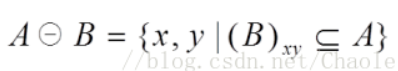1. 用结构元素，扫描图像的每一个像素

2. 用结构元素与其覆盖的二值图像做“与”操作

3. 如果都为1，结果图像的该像素为1。否则为0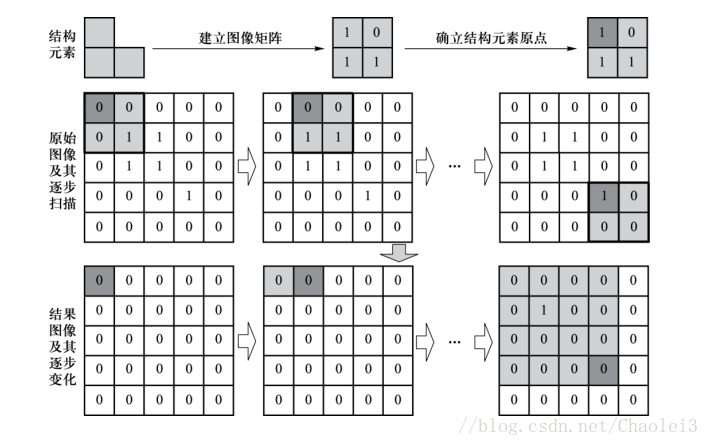Mat ErosionTransform(Mat &src,int kernelSize)
{
Mat dst(src.rows, src.cols, src.type(), Scalar(0));
Mat kernel = Mat::ones(kernelSize, kernelSize, CV_8UC1);
if (src.channels() == 1) {
int rowsSub = int(kernel.rows / 2);
int colsSub = int(kernel.cols / 2);
for (int i = rowsSub; i < src.rows-rowsSub; i++) {
for (int j = colsSub; j < src.cols-colsSub; j++)
{
int flag = 0;
for (int ki = 0; ki < kernel.rows; ki++)
{
for (int kj = 0; kj < kernel.cols; kj++)
{
int i_ = i+ki - rowsSub;
int j_ = j+kj - colsSub;
if (src.at<uchar>(i_, j_) == 0 && kernel.at<uchar>(ki, kj) >0)
flag = 1;
}
}
if(!flag)
dst.at<uchar>(i, j) = 255;
else
dst.at<uchar>(i, j) = 0;
}
}
}
return dst;
}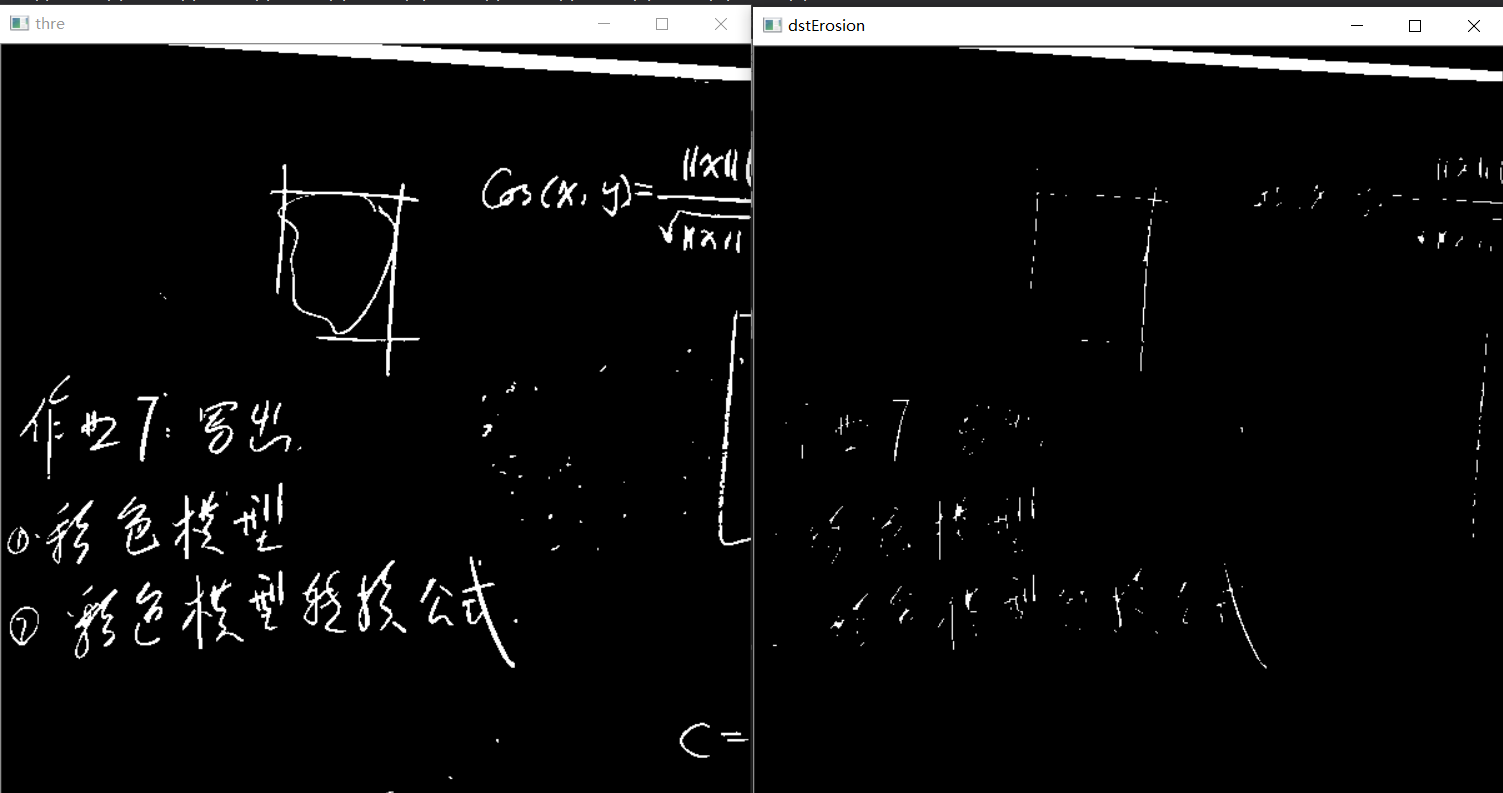### 膨胀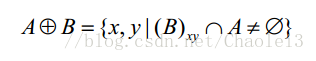1. 用结构元素，扫描图像的每一个像素

2. 用结构元素与其覆盖的二值图像做“与”操作

3. 如果都为0，结果图像的该像素为0。否则为1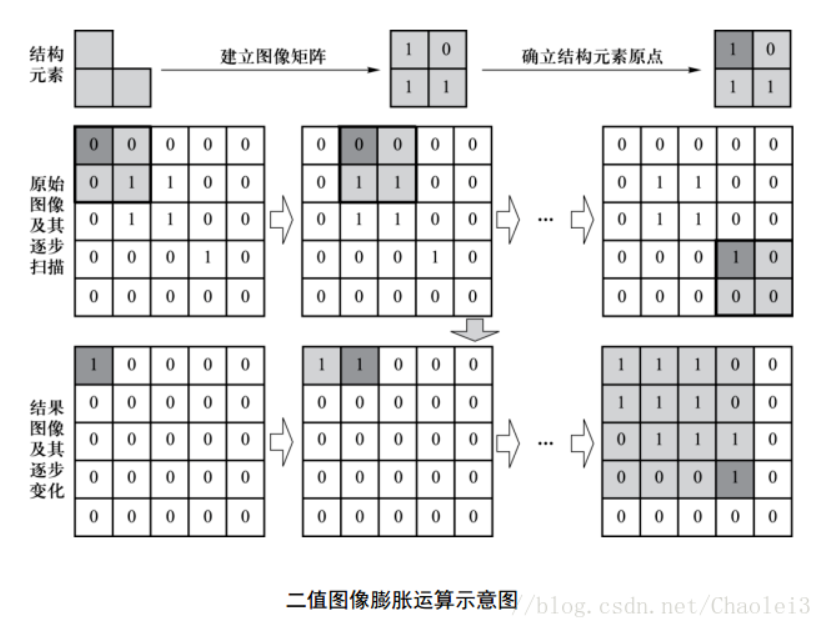Mat DialateTransform(Mat &src, int kernelSize)
{
Mat dst(src.rows, src.cols, src.type(), Scalar(0));
Mat kernel = Mat::ones(kernelSize, kernelSize, CV_8UC1);
if (src.channels() == 1) {
int rowsSub = int(kernel.rows / 2);
int colsSub = int(kernel.cols / 2);
for (int i = rowsSub; i < src.rows - rowsSub; i++) {
for (int j = colsSub; j < src.cols - colsSub; j++)
{
int flag = 0;
for (int ki = 0; ki < kernel.rows; ki++)
{
for (int kj = 0; kj < kernel.cols; kj++)
{
int i_ = i + ki - rowsSub;
int j_ = j + kj - colsSub;
if (src.at<uchar>(i_, j_) >0 && kernel.at<uchar>(ki, kj) >0)
flag = 1;
}
}
if (!flag)
dst.at<uchar>(i, j) =0;
else
dst.at<uchar>(i, j) = 255;
}
}
}
return dst;
}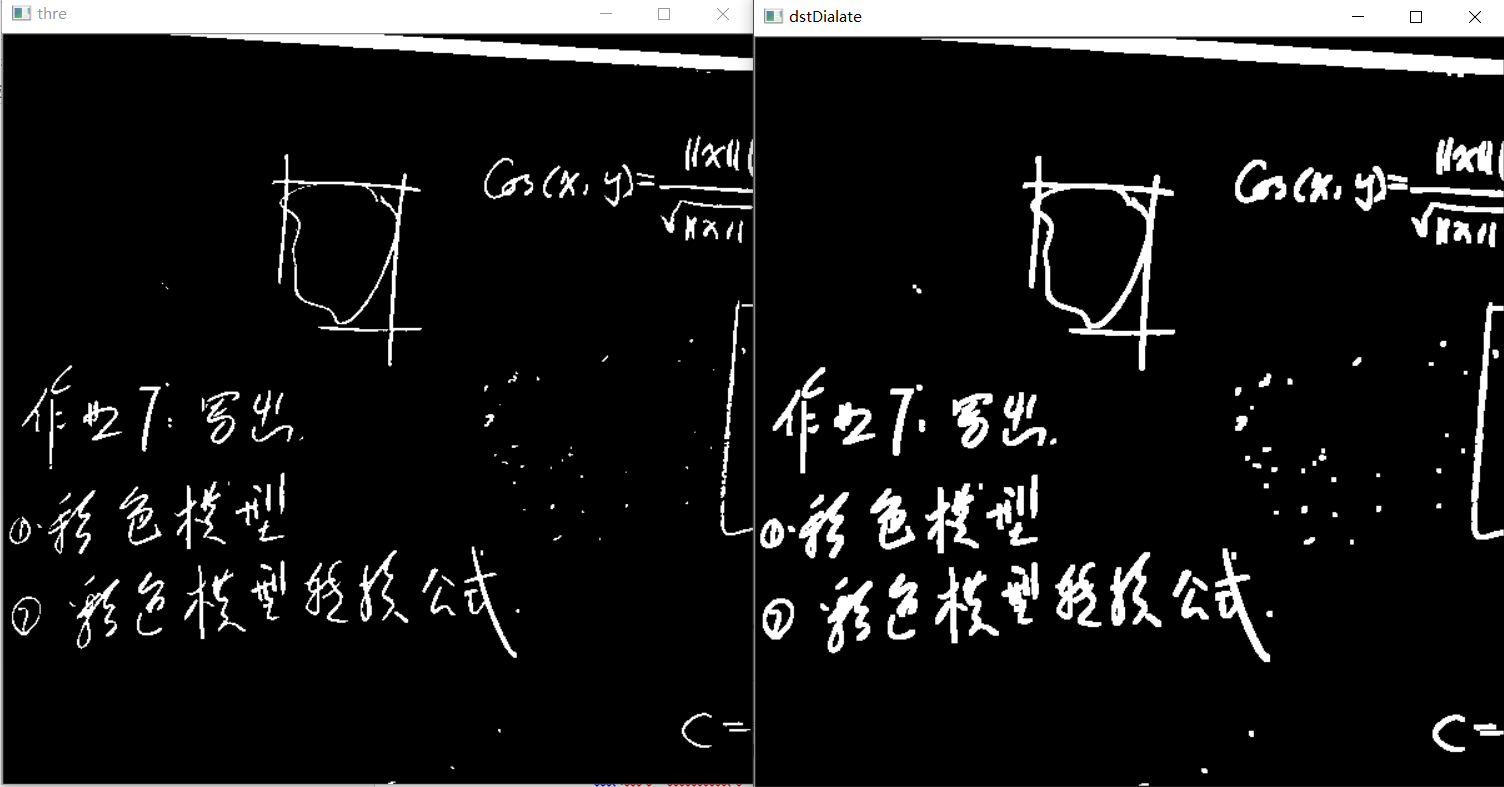### 闭运算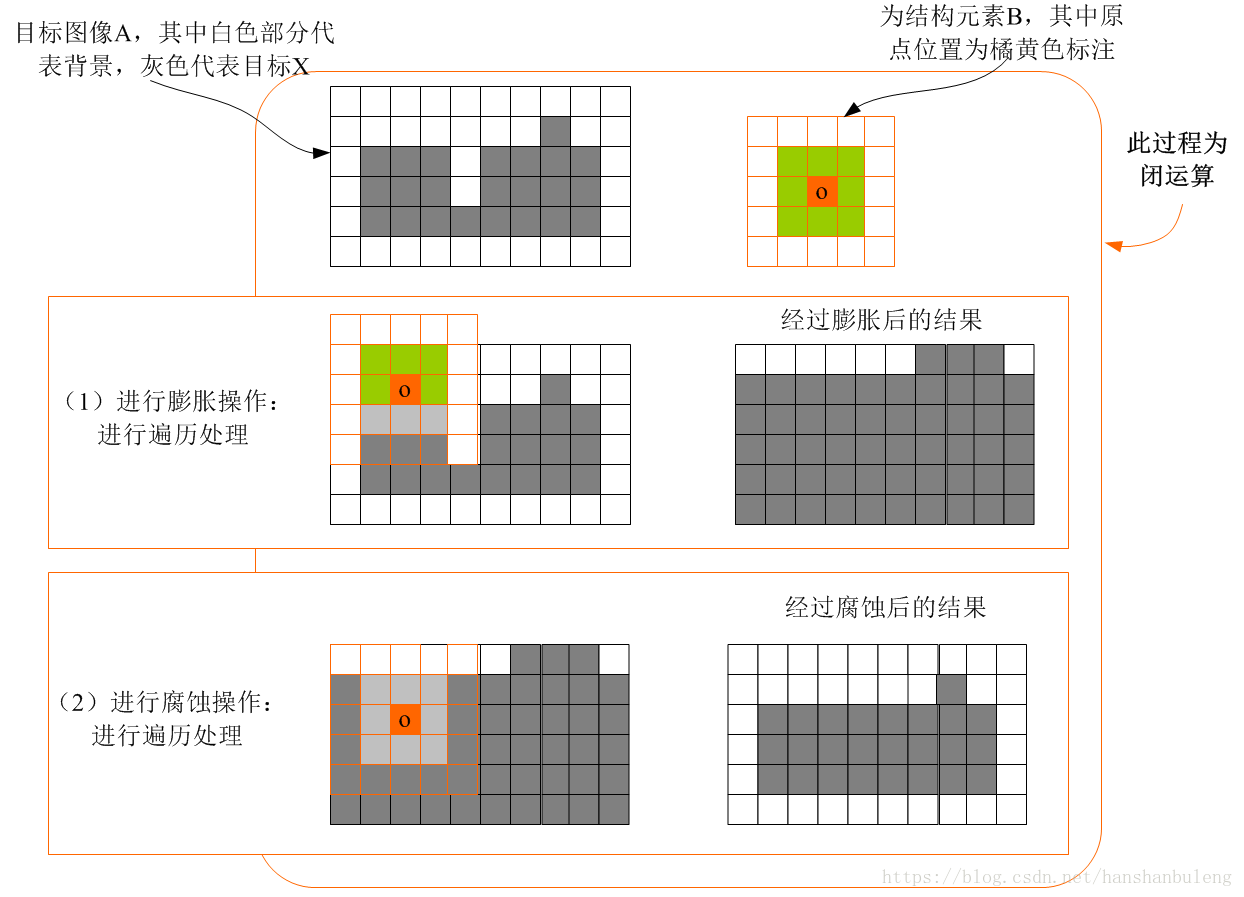Mat ClosingTransform(Mat &src, int kernelSize)
{
Mat dialateImg = DialateTransform(src, kernelSize);
return ErosionTransform(dialateImg, kernelSize);
}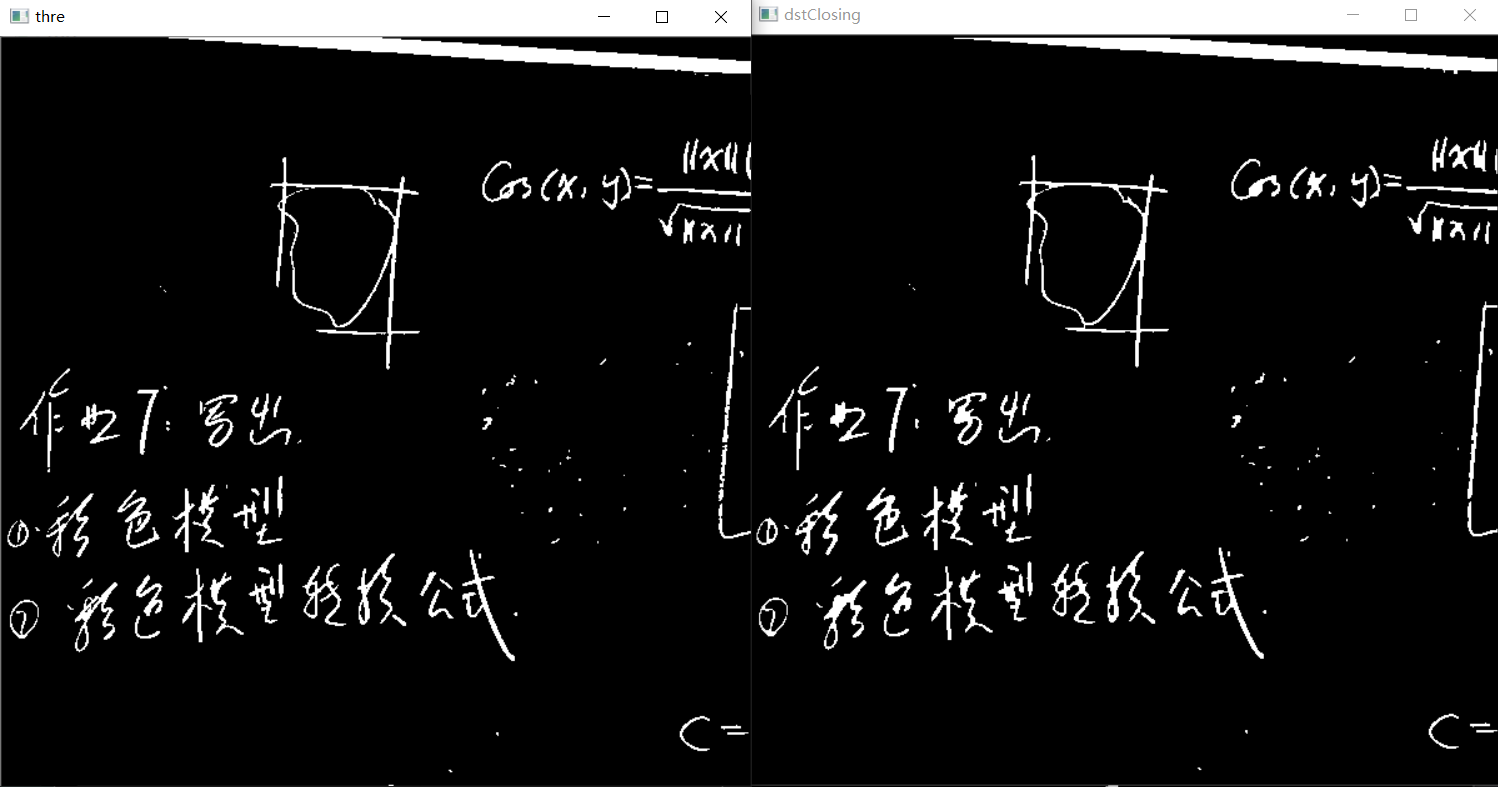### 开运算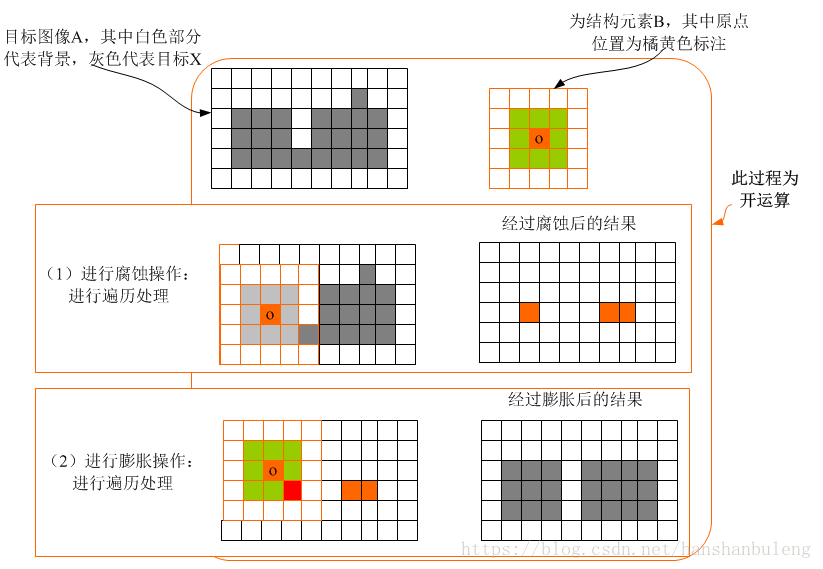Mat OpeningTransform(Mat &src, int kernelSize)
{
Mat erosionImg = ErosionTransform(src, kernelSize);
return DialateTransform(erosionImg, kernelSize);
}

kernelSize=5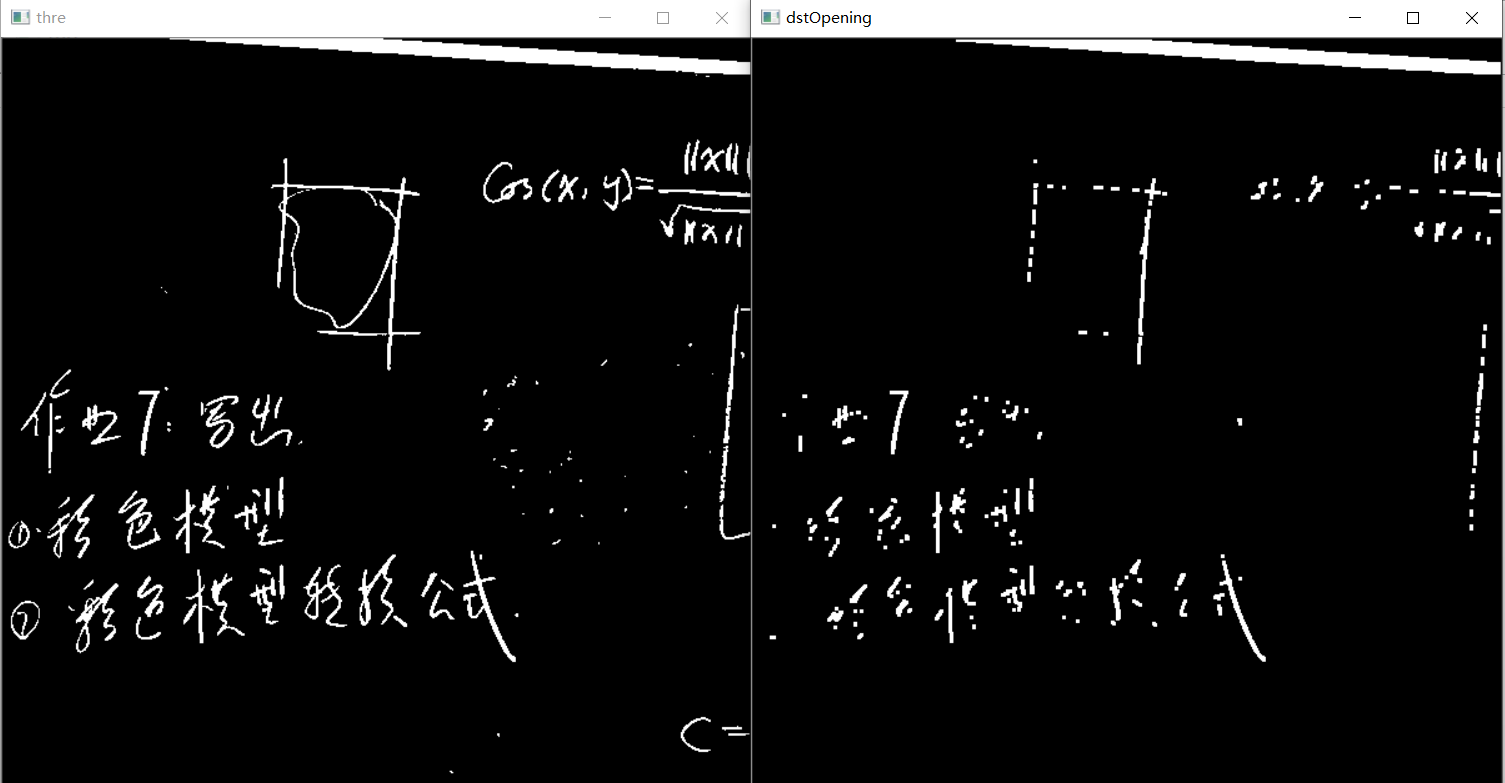CSDN博客：chaolei_9527. 数学形态学运算——腐蚀、膨胀、开运算、闭运算.https://blog.csdn.net/chaolei3/article/details/79618602.2018-03-20

CSDN博客：青雲-吾道乐途. 膨胀与腐蚀的彻底击破.https://blog.csdn.net/qq_37059483/article/details/77878829.2017-09-07

CSDN博客：HanShanBuLeng. 形态学应用——图像开运算与闭运算.https://blog.csdn.net/hanshanbuleng/article/details/80657148.2018-06-11

2017-07-27 20:52:45 u010460650 阅读数 2686
• ###### OpenCV3.2 Java图像处理视频学习教程

OpenCV3.2 Java图像处理视频培训课程：基于OpenCV新版本3.2.0详细讲述Java OpenCV图像处理部分内容，包括Mat对象使用、图像读写、 基于常用核心API讲述基本原理、使用方法、参数、代码演示、图像处理思路与流程讲授。主要内容包括opencv像素操作、滤波、边缘提取、直线与圆检测、形态学操作与分水岭、图像金子塔融合重建、多尺度模板匹配、opencv人脸检测、OpenCV跟Tomcat使用实现服务器端图像处理服务。

4125 人正在学习 去看看 贾志刚

$~~~~~~$形态学图像处理是指从图像中提取一些“内涵”，也就是一些想得到的属性。形态学处理的数学工具是集合论，在冈萨雷斯的书中研究对象以后两种一种是二值图像，一种是灰度图像。
$~~~~~~$在在二值图像中中主要有膨胀和腐蚀两种基础操作，大多数形态学算法都是基于膨胀和腐蚀这两种。不管那种操作都需要用到一种处理的工具，这种工具定义了如何对图像进行腐蚀，在图像处理中这种工具被称为结构元（structure element），就相当与一种探测器，探测到的部分都要进行腐蚀或者膨胀，不同的结构元对同一种图像处理的结果不同。

## 1.二值图像操作

### 1.1腐蚀与膨胀

$~~~~$利用结构元对图像进行腐蚀，对图像进行探测，如果结构元探测的区域内包含所有的前景色，那么就用前景色像素点替换中心位置的点（或者说不作改变），如果结构元探测的区域内包不全包含前景色，那么就用背景色取代该点（或者说做出取反的改变）。

### 1.2开操作与闭操作

$~~~~$开操作是指先腐蚀后膨胀，容易消去一些较小的细节。如断开较细的连接，消除细的突出物。有一定的平滑效果。
$~~~~$闭操作是指先膨胀后腐蚀，容易弥补一些较小的细节。如弥合较窄的间断，消除较小的孔洞。

### 1.3击中或击不中变换

$~~~~$这是一个形状检测的一个工具，旨在用结构元去图像中检测与结构元结构完全一致的图像。

## 2一些基于二值图像的节本操作

### 2.1边界提取

$~~~~~~$原图像-腐蚀过后的图像产生边界

### 2.2孔洞填充

$~~~~~~$条件膨胀

### 2.3提取连通分量

$~~~~~~$条件膨胀

### 2.4 凸壳

$~~~~~~$利用有凸性特质的结构元组对图像进行有条件的膨胀，最后达到凸形

### 2.9形态学重建

• 侧地膨胀和腐蚀
$~~~~~~$利用模版对标记图像进行有约束的膨胀或者腐蚀，这里的模板图像就像是一个限定器，按照这个模板对图像进行条件的膨胀或者腐蚀。
• 重建开操作与重建闭操作

开操作中，腐蚀会删除较小的物体，而膨胀会恢复一流物体的形>状，然而恢复的准确性高度依赖物体的形状和所用结构员的相似性，但重建开操作可以正确恢复腐蚀后的物体形状。 — 《数字图像处理》 冈萨雷斯

$~~~$ 之所以能够比较正确的恢复腐蚀后的物体形状，这是因为在腐蚀之后有测地膨胀，测地膨胀可以依靠原图像作为模板来进行条件膨胀，从而达到与原图想一致的效果。
• 填充空洞
• 边界清除

## 3灰度级形态学

### 3.1膨胀与腐蚀

$~~~~~$与二值图像不一样，灰度级的图像再利用SE(结构元)进行腐蚀或者膨胀时，使用的最大值灰度和最小值灰度替代。用SE探测区域，膨胀用最大值（最亮的点）替换中心点，腐蚀是用最小值（最暗的点）替换中心点。

### 3.2开操作和闭操作

$~~~~~$开操作就是先腐蚀后膨胀，灰度级开操作能让图像的亮点变得平滑，也就是变暗。灰度级闭操作能让图像的暗点变得更平滑， 也就是变得额更亮。有变化的点一般比结构元小。

## 4一些基于灰度级图像的算法操作

### 4.1形态学平滑

$~~~~~$利用灰度级开操作抑制亮点和闭操作抑制暗点的操作对图像进行平滑处理

### 4.2形态学梯度

$~~~~~$膨胀减去腐蚀得到边缘/轮廓（就是变化幅度比较的大的那部分）

### 4.3顶帽变化和底帽变化

$~~~~~$顶帽变化的名字很形象化，开操作会抑制亮的区域（这个区域比结构元小），在灰度级图像的三维表达中（灰度的大小为Z轴，坐标为XY轴），表现为一个平滑的凸曲线被消去了一部分，消去的这部分像一个帽子，所以就叫做顶帽变换，我猜的哈哈。底帽同理。其实就是来求消去的图像亮区域。

### 4.4粒度检测

$~~~~~$可以用来判断图像中颗粒尺寸分布的领域。如可以利用一组逐级增大的结构元（这些结构员与颗粒应该是具有相似性的）进行一次次的顶帽*（或者底帽）变化，这时的“帽子”大小就与消除的颗粒大小具有某种联系喽。

### 4.6灰度级形态学重建

• 侧地膨胀和侧地腐蚀
$~~~~$利用模版对图像进行有约束的膨胀和腐蚀。这里的模版图像用来限定操作的结果。

• 重建开操作和重建闭操作

• 重建顶帽和重建底帽
• -

2019-10-03 17:00:32 weixin_41424926 阅读数 309
• ###### OpenCV3.2 Java图像处理视频学习教程

OpenCV3.2 Java图像处理视频培训课程：基于OpenCV新版本3.2.0详细讲述Java OpenCV图像处理部分内容，包括Mat对象使用、图像读写、 基于常用核心API讲述基本原理、使用方法、参数、代码演示、图像处理思路与流程讲授。主要内容包括opencv像素操作、滤波、边缘提取、直线与圆检测、形态学操作与分水岭、图像金子塔融合重建、多尺度模板匹配、opencv人脸检测、OpenCV跟Tomcat使用实现服务器端图像处理服务。

4125 人正在学习 去看看 贾志刚

# 内容简介

本篇博客介绍的形态学图像处理操作。形态学在数学语言里是集合论。在图像处理中，形态学是很重要的分支，但是它又不像傅里叶变换等内容，包含详细的公式推导。通常形态学操作的数学表达都比较抽象，不太容易理解。因此，本篇博客打算以文字与实践相结合的方式进行阐述。
本篇文章内容中，所有具有处理结果图像的形态学操作均是本人根据教材自己编写的代码，由于代码较多，之后会放在个人github中，如在公布之前有需要的读者，可以留言说明。
本篇内容主要知识和相关操作图片来自冈萨雷斯第三版的《数字图像处理》，如对细节内容感兴趣的读者可以查阅相关书籍进行参考。本篇博文的内容与原书内容的顺序有所出入，如有不足欢迎留言指正，谢谢。

# 1. 预备知识——结构元

其实结构元(SE,Structure Element)最直接的理解就是卷积操作中的卷积核，或者是空间域滤波中提到的滤波器。虽然形态学操作中结构元的形状可以是任意的，但是由于在图像操作中，为了方便计算，通常要求结构元是矩形的阵列，对于任意形状的结构元，如果不满足矩形的要求，则用0将其填充为矩形即可。
另外，结构元内部的有效元素不像滤波器那样有权值，通常结构元中只分为两种元素，就是0和1，不会出现其他数值的系数。(当然对有些算法来说也有例外)。结构元对图像进行的操作也和卷积非常类似，就是由结构元的中心依次滑过图像，然后进行设计好的操作即可。接下来就开始介绍图像处理中常见的形态学操作。

# 2. 形态学基本操作

形态学基本操作是形态学最重要的基础，几乎所有复杂的形态学操作都由基本形态学操作组合而成。在本章节中，主要介绍的是二值图像的形态学操作，因此本章中默认图像都是二值图像，且由0表示背景像素，1表示前景像素。
下面结合集合论的数学表达以及实际使用案例对各种操作进行介绍。

## 2.1 腐蚀和膨胀

1).腐蚀(erode)
$A\ominus B=\{z|(B)_z\subseteq A\} \Leftrightarrow A\ominus B=\{z|(B)_z\cap A^c=\empty\}$
其中，$A^c$是图像A的补集，即1-A。上式表达的意思就是，在z的移动下，如果结构元B能够完全包含在图像A中(完全包含意味着结构元B中的有效元素对应于图像A中的位置全都是前景像素。z的移动就是针对于图像A的所有像素范围的移动。上图中，左边实线围住的9x9方格就是结构元B，红色代表其有效区域，白色代表其无效区域(0填充)。右侧实现代表图像A，浅蓝色部分代表A的前景像素，白色代表背景像素。当结构元滑动到1区域时，所有有效区域对应的像素都是前景像素(紫色区域,红+蓝)，因此称此时$B\subseteq A$，此时，1号位置在结果图像中应该置1。反之，如果只有部分区域重合，如结构元处于2号位置，那么说明此时的2号点不满足腐蚀条件，应该在结果图像中置0。
下面是腐蚀操作对实际图像的处理效果。

• 第一张为原图。
• 第二张为用11x11的全1模板作结构元进行的腐蚀，可以看到，由于细线的宽度小于11像素，所以细线不可能完全包含SE，因此细线部分被“腐蚀”。
• 第四张图结构元尺寸为15x15，可以看到，连最粗的线都被腐蚀了。
• 第五张SE尺寸为45x45，就可以将除了中心方块以外的所有元素腐蚀掉。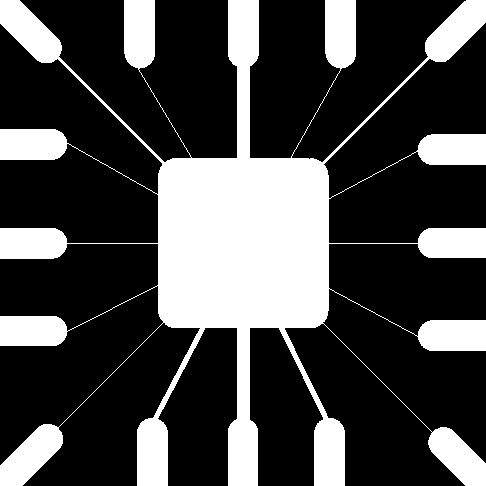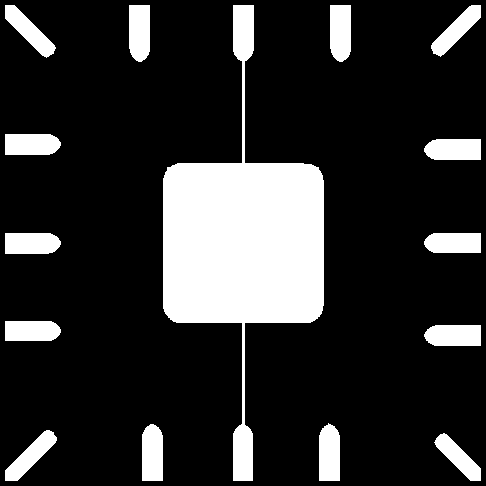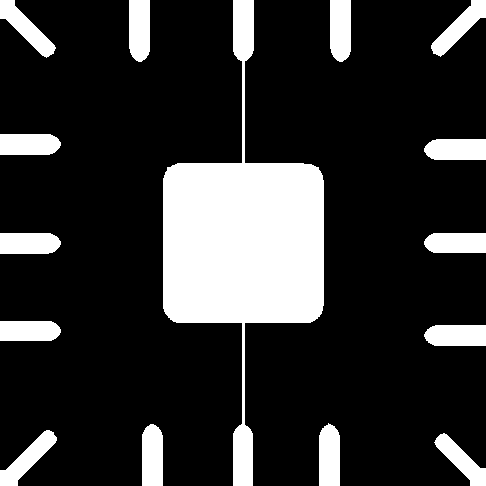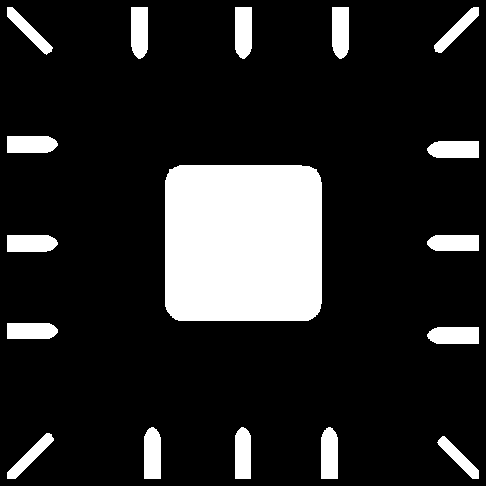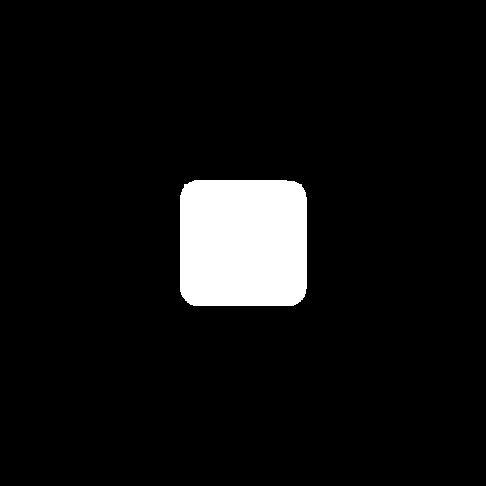2).膨胀(dilate)
$A\oplus B=\{z|(\hat B)_z\cap A\ne\empty\} \Leftrightarrow A\oplus B=\{z|[(\hat B)_z\cap A]\subseteq A\}$
其中，$\hat B$称为结构元B的反射，指的是，结构元B绕结构元的中心(通常设置在SE矩阵中心)旋转180°后的新结构元。对于对称的结构元，就有$B=\hat B$
膨胀的集合含义就是，SE和图像的前景像素至少有一个交点，那么当前移动到的像素就置1，否则置0。在膨胀的条件下，之前图示中的1,2号区域均会被置1。(腐蚀时，2号区域是被置0的)。
下面是膨胀操作对实际图像的处理效果。

• 第一张图是原图，可以看到原图中的字母多数都有断裂现象，这里需要着重观察右下角的“year"单词；
• 第二张图是opencv自带的cv2.dilate函数，用SE尺寸3x3的核得到的结果。可以看到，膨胀后，所有字母都变粗了(膨胀了)，大部分断裂的区域都连接上了。但是这和教材中的处理结果略有不同，因为opencv提供的膨胀操作，不支持自定义结构元，其结构元是3x3的全一矩阵。而教材中使用的SE是3x3的四个角为无效元素的十字型结构元。
• 第三张图是自己编写的dilate函数，结构元也使用和教材中一模一样，因此结果是和教材里一样的。注意观察可以发现，图3相比于图2膨胀程度更高，因此，"year"字母中的"ea"的断裂修复效果也比图2更好。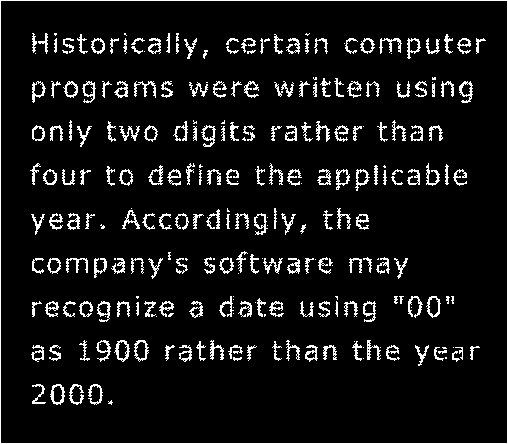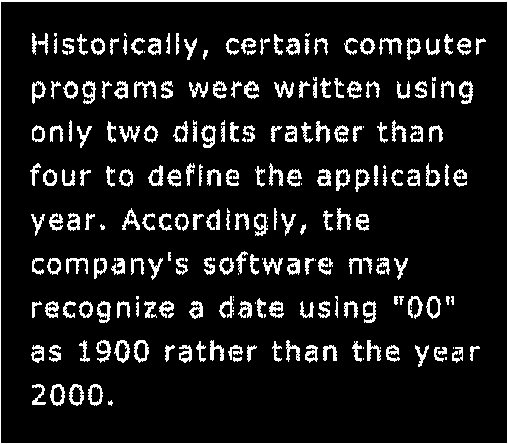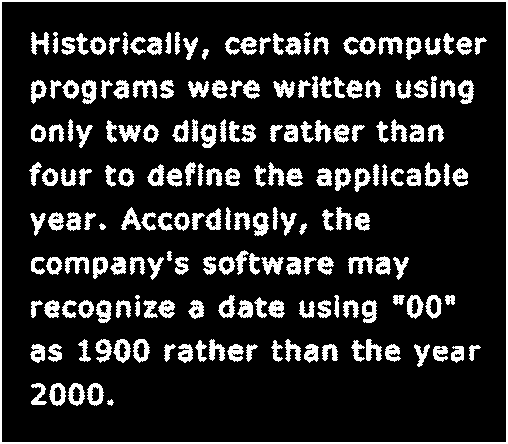3).腐蚀和膨胀的对偶性

• $(A\ominus B)^c=A^c \oplus \hat B$
• $(A\oplus B)^c=A^c \ominus \hat B$

## 2.2 开操作与闭操作

开操作和闭操作都是基于腐蚀和膨胀操作的。
1).开操作(opening)
$A\circ B=(A\ominus B)\oplus B$
开操作就是先用SE腐蚀图像A，再对腐蚀后的结果进行膨胀。第一反应或许会觉得，这操作不是很多余吗？其实细想后发现，其中自有妙处。假设图像中存在很多细小琐碎的颗粒或噪声，那么经过第一步腐蚀后，这些细小的噪声就会被腐蚀而消失，尽管紧接着进行了膨胀操作，但是由于细小颗粒已经完全消失，因此即使膨胀也无法恢复。相反，那些图像中的主体，经过腐蚀后仍然存在，再经过膨胀就可以恢复原样。这么一说，开操作是不是非常有用呢？仔细理解形态学操作的含义非常重要，虽然形态学操作单独拆开看都不是很复杂，但是要在应用中发挥最巧妙的效果，需要使用者对形态学各种操作能得到的效果进行深入理解。否则只会弄巧成拙或完全无法发挥形态学图像处理的威力。
开操作的性质：

• $A\circ B$是A的一个子集(子图像)；
• 如果C是D的一个子集，则$C\circ B$$D\circ B$的一个子集；
• $(A\circ B)\circ B=A\circ B$

以下是开操作的实际操作效果：

• 图1是原图像，可以看到，在采集指纹的图像中，存在很多不需要的噪声干扰；
• 图2是使用opencv的cv2.morphologyEx函数进行的图像开操作，其效果和教材中的开操作效果出入较大，猜测原因是opencv提供的形态学函数只支持输入结构元的尺寸(kernel)，但是具体该参数如何生成结构元并未说明。所以具体原因需要阅读opencv源码后再进行分析，如果看完源码知道原因了会及时在这里更新。有知道的读者也可以留言说明。因此，这也说明了，不懂得原理没有自己实践实现过算法，只会调用第三方库是很危险的。因为你不理解别人的库中算法是如何实现的，和你实际想要的效果是否一致。因此，对于第三方库，源码阅读是非常必要的。
• 图3是根据之前编写的腐蚀、膨胀操作代码实现的开操作，可以看到，这个效果和教材中是一模一样的，开操作使得细小的噪声完全消失了，而指纹主体也通过膨胀很好的恢复了。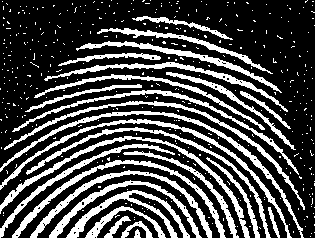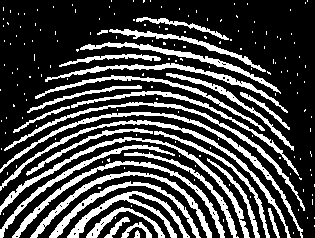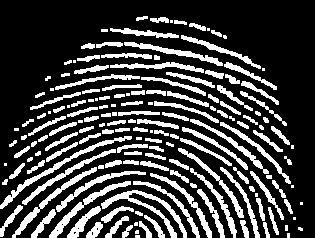2).闭操作(closing)
$A\bullet B=(A\oplus B)\ominus B$
闭操作和开操作正好相反，是先膨胀再腐蚀。如果图像中存在细小的孔洞或者缝隙，通过膨胀后就可以填充细小孔洞和缝隙，使其和主体连接起来，而再腐蚀的时候，只会腐蚀图像主体，而不会再恢复缝隙等瑕疵。因此，闭操作很适合拿来修补图像瑕疵的。
闭操作的性质：

• A是$A\bullet B$的一个子集(子图像)；
• 如果C是D的一个子集，则$C\bullet B$$D\bullet B$的一个子集；
• $(A\bullet B)\bullet B=A\bullet B$
以下是闭操作的实际操作效果：
• 下图是在开操作得到的图3基础上进一步进行闭操作的结果图，可以看到，相比于之前的图像，闭操作将很多指纹中断裂的区域连接起来，使得指纹的图像更加完整。但是对于较大的裂缝，闭操作是无能为力的，当然你可以增大SE的尺寸，但是这样很可能使得指纹不同纹路也连接起来，反而得不偿失。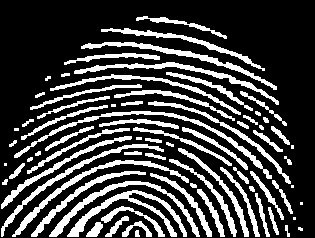3).开操作和闭操作的对偶性
• $(A\bullet B)^c=(A^c\circ \hat B)$
• $(A\circ B)^c=(A^c\bullet \hat B)$

## 2.3 击中和击不中变换(hit and miss transform)

这个基础形态学变换就没有之前几个那么直观了。理解起来比较抽象。首先，假设有个结构元$B_1$$B_1$的有效元素共同组成了特定的形状。其次，设W是一个比$B_1$尺寸更大的全1模板，记$B_2=W-B_1$，即从模板W中将$B_1$挖去。然后，击中和击不中变换就可以表达为：
$A\circledast B=(A\ominus D)\cap[A^c\ominus(W-D)]=(A\ominus B_1)\cap (A^c\ominus B_2)$
击中和击不中变换的直观理解
假设有图像A(如下图1)，$A=C\cup D\cup E$，表示A图像中有C,D,E三个前景子区域。如果我们想要在图像A中寻找是否存在形状D(图1中的45x45的正方形)，怎么办？这时候，我们就可以将结构元$B_1=D$，然后选择合适的W求出$B_2=W-D$，对图像进行击中和击不中变换，变换所得到的结果，如果A中存在和D一样的形状，则会留下一个点，该点是D形状所在位置的中心；如果不存在D，那么得到的图像就是空。
其中，击中和击不中变换中的$A\ominus B_1$是在图像中找到与$B_1$匹配的结果，即击中结果，如下图2。注意，击中并不代表说A图像中有与$B_1$一模一样的形状，比如左侧长方形，其中包含形状D，但是并不与D完全相同。所以才需要进一步进行击不中变换。而$A^c\ominus B_2$中的$B_2$可以理解为一个框架的中间掏出一个与D形状一模一样的空缺(见下图5，黑色区域为45x45,白色区域为47x47），框架的白色区域通常设置为1个像素宽，图中为了显示而故意夸大了。然后将该框架试图通过图像A，白色区域表示实体，框架的实体和图像中的实体实会相互阻隔而无法通过的。因此可以看到，图像A中只有比形状D小的实体可以穿过$B_2$，这些$B_2$能穿过的地方的集合，就叫做击不中区域，如下图3中的白色区域，就代表框架$B_2$的中心对准白色区域任意点时，框架可以穿过图像(或者说图像A中的实体击不中框架)。
综上所述，将击中结果和击不中结果相交，最终所得到的结果为下图4。相交的结果直观点可以理解为，既可以被D形状完全击中，又可以被以D形状为空的框架完全穿过的实体，那就肯定只能是和D形状一模一样的实体。图4中可以看到一个白点，这就说明图像A中存在与形状D一模一样的区域，且该区域中心的位置就是图4白点所在位置。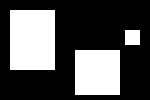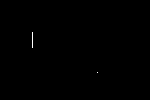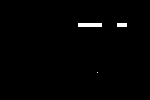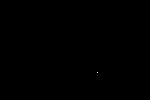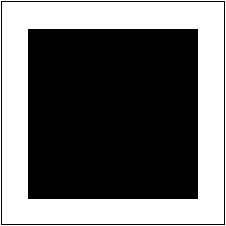击中和击不中变换更常用的场景——击中变换
在某些场合中，我们可能对检测某个集合内由1和0组成的特定模式感兴趣(这种情况下，要求结构元中的1对于图像的前景元素，结构元中的0对应图像的背景元素，结构元中的x则表示对该区域不感兴趣，可以为任意元素)，此时，击中与击不中变换就不需要击不中变换(因为前景和背景都属于我们感兴趣的范围)，而简单地转换成了特定模式的腐蚀操作。(和原始的腐蚀不同，原始的腐蚀的结构元中，结构元中为0的部分相当于这里的x，它不要求对于的图像像素一定是背景元素，而可以是任意元素)。
以下图为例，假设此时的结构元为下图左的样子，其中红色为1区域，灰色为0区域，x为不感兴趣区域。那么，此时图像要匹配，则需要满足SE的红色区域对应的图像都为前景像素，灰色区域对应的图像均为背景像素，而x区域对应的图像则不感兴趣，不做要求。如果满足上述条件，则将当前位置的结果图像置1，或者说是结构元SE击中图像/在图像中找到了匹配。下图中间的实线区域代表图像。下图右侧的实线区域中，1号位置表示在图像中找到了SE的匹配；而2号区域，中间正上方和正下方不满足需要为背景图像的要求，因此该位置并未找到匹配。# 3. 基本的形态学算法

有了形态学的基础操作，就可以将其进行组合得到基本的形态学算法，以下一一介绍。同样，下述算法都是基于二值图像的操作。基于灰度图像的形态学算法之后还会介绍。

## 3.1 二值图像边缘提取

$\beta(A)=A-(A\ominus B)$
其中，$\beta(A)$是提取出来的A图像的边缘图像；B是适当的结构元，如果B尺寸比较大，那么通常提取到的边缘就会比较粗。稍微观察一下就会理解，二值图像的边缘其实就是图像A被腐蚀掉的部分。因此只要腐蚀前和腐蚀后的图像相减，就可以得到边缘。具体效果见下图：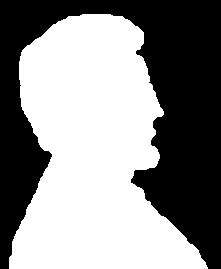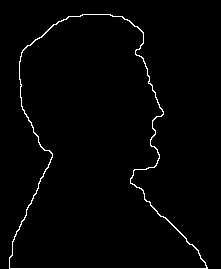## 3.2 孔洞填充

算法步骤：

• 首先，确认图像孔洞中的任意一个像素，将其标记为1，注意，如果有多个孔洞，则对于要填充的每个孔洞，都需要一个孔洞内部的任意像素。将其他位置都标记为0，作为迭代初始阵列$X_0$$X_0$的尺寸和标记孔洞的像素位置都要和原图像需保持一致。
• 选择合适的结构元B，比如下图中的两种都可以。• 进行如下迭代过程：$X_k=(X_{k-1}\oplus B)\cap A^c,k=1,2,...$
• 直到$X_K=X_{K-1}$则停止迭代。此时，$dst=A+X_K$就可以得到孔洞填充后的图像，其中$X_K$是将孔洞转换为前景像素后的图像。

为什么迭代公式中要存在" $\cap A^c$ "这一步？这称为“条件膨胀”,有兴趣的读者可以试试去掉这一步，那么最终的孔洞膨胀的结果将会填满整个图像，得到一张全白的图。通过与$A^c$进行交集的操作，保证我们膨胀的过程中，$X_k$图像始终不会将原图像的前景图像覆盖，也就是用原图像的前景像素限制了膨胀的空间，所以才叫条件膨胀。
下面是对图像进行孔洞填充的示意图，左图为原图，右图为填充后的结果。中间的动图是填充过程。我只取了上半部分的孔洞进行填充，所以最终并没有把所有孔洞都填满(初始化标记孔洞位置太麻烦了)。另外，可以看到，其中有个孔洞的中间是有个白点的，而在填充时这个白点也没有被填(动图中的小黑点)，所以可以从中理解条件膨胀的含义。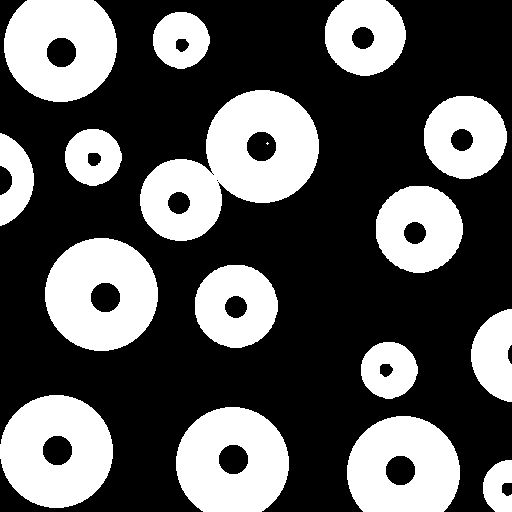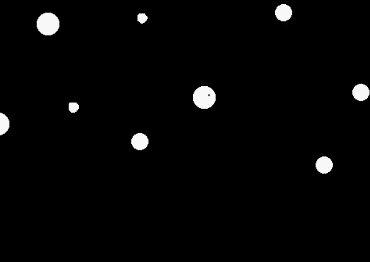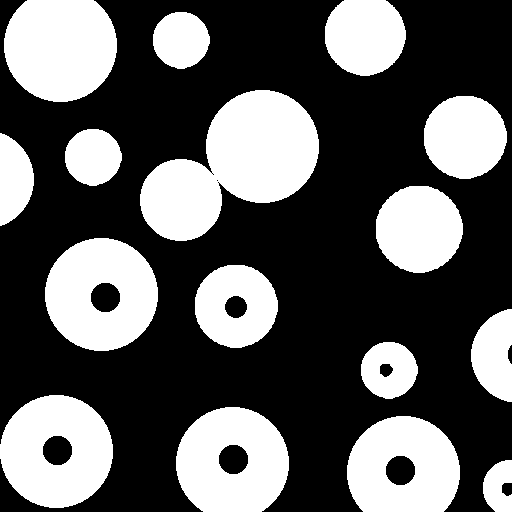## 3.3 连通分量的提取

其实算法和孔洞填充一样，只是第一步初始化的时候，选择的标记像素点是想要提取的连通分量中的任意一点。第三步迭代的时候，条件膨胀从A的补集替换为A本身。这是很直观的，因为我们现在想要提取的是图像前景，因此需要将膨胀的元素限制在图像前景范围内。
算法步骤：

• 首先，确认图像连通分量中的任意一个像素，将其标记为1，注意，如果有多个连通分量，则对于要提取的每个分量，都需要一个连通分量内部的任意像素。将其他位置都标记为0，作为迭代初始阵列$X_0$$X_0$的尺寸和标记连通分量内部像素的位置都要和原图像需保持一致。
• 选择合适的结构元B，比如下图中的两种都可以。• 进行如下迭代过程：$X_k=(X_{k-1}\oplus B)\cap A,k=1,2,...$
• 直到$X_K=X_{K-1}$则停止迭代。此时，$dst=X_K$就可以得到提取的连通分量。

如果你只想要提取图中某个连通区域，不希望提取其他区域，并且可以确认该连通区域一定会覆盖图像中某一点，那么这种算法可以很好地完成任务，提取想要的连通部分。
连通分量的提取实践如下，使用的原图像是孔洞填充的原图像，只提取了其中两个较小的圆环：## 3.4 凸壳(convex hull)

定义：如果集合A内任意两个点的直线段都在A的内部，则称集合A是凸形的。任意集合S的凸壳H是包含S的最小凸集。差集H-S称为S的凸缺
算法步骤

• 设原图像为A，首先，确定算法中需要使用的四种结构元，如下图。• 对于每个结构元$B^i,i=1,2,3,4$，均作如下迭代：
• $X_0^i=A$
• $X_k^i=(X_{k-1}\circledast B^i)\cup X_{k-1},\quad k=1,2,3...$
• 直到$X_K^i=X^i_{K-1}$，记录$D^i=X_K^i$
• 最终，得到图像A的凸壳为：$C(A)=\bigcup_{i=1}^4 D^i$

上述过程有两点说明，均很重要！
1). 算法中的 $\circledast$仅包含击中变换的击中和击不中变换，关于什么是仅包含击中变换，可以参考2.3节最后的解释；
2). 原《数字图像处理》教材中，迭代公式是 “$X_k^i=(X_{k-1}\circledast B^i)\cup A$” ，经验证后发现是错的，迭代公式应该是 “$X_k^i=(X_{k-1}\circledast B^i)\cup X_{k-1}$” 。读者也可自行验证。

上述算法有一个明显的缺点，就是求出的凸壳可能不是包含原图像的最小凸集。优化最终结果有个常用的办法，就是让求出的凸壳不超过初始点集在水平和垂直方向上的尺寸。当然，也可以针对求出的结果进行进一步的限制生长，但是通常会损失算法效率，需要慎重考虑。
凸壳算法的具体实践如下图，图1是原始图像，图2动图是整个求解凸壳的过程，动图可按2行3列观看，其中第一行第一列是$B^1$的迭代过程，第二行第一列是$B^2$的迭代过程，第一行第二列是$B^3$的迭代过程，第二行第二列是$B^4$的迭代过程，第一行第三列是每次迭代结束后叠加在一起的$C(A)$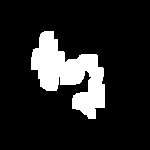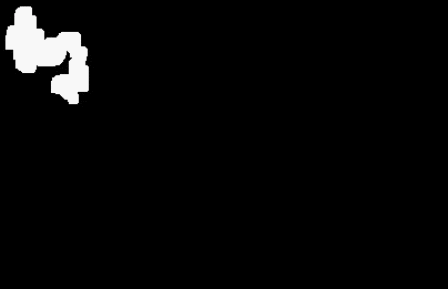2017-01-13 17:22:59 rongfzh1990 阅读数 835
• ###### OpenCV3.2 Java图像处理视频学习教程

OpenCV3.2 Java图像处理视频培训课程：基于OpenCV新版本3.2.0详细讲述Java OpenCV图像处理部分内容，包括Mat对象使用、图像读写、 基于常用核心API讲述基本原理、使用方法、参数、代码演示、图像处理思路与流程讲授。主要内容包括opencv像素操作、滤波、边缘提取、直线与圆检测、形态学操作与分水岭、图像金子塔融合重建、多尺度模板匹配、opencv人脸检测、OpenCV跟Tomcat使用实现服务器端图像处理服务。

4125 人正在学习 去看看 贾志刚# 1. 腐蚀 Erosion

AB={z|(B)zA}$A\circleddash B= \{z|(B)_z\subseteq A \}$
B$B$移动z$z$单位(B$B$的中心在z$z$)后, 仍然包含在A$A$中. 那么z$z$的集合就是B$B$A$A$的腐蚀.

AB={z|(B)zAc=}$A\circleddash B= \{z|(B)_z \bigcap A^c =\varnothing \}$

# 2. 膨胀 Dilation

AB={z|(B^)zA}$A\bigoplus B= \{z|(\hat{B})_z \bigcap A \neq \varnothing \}$
B$B$的镜像原点在z$z$, 和A$A$至少有一点的交集.

# 3. 开运算 Opening

AB=(AB)B$A \circ B=(A\circleddash B)\bigoplus B$

# 4. 闭运算 Closing

AB=(AB)B$A \cdot B=(A\bigoplus B)\circleddash B$

# 5. Hit-or-Miss

AB=(AD)[Ac(WD)]$A\circledast B=(A\circleddash D)\bigcap[A^c\circleddash (W-D) ]$
B表示包含目标D及其背景的集合.

# 6. 边界提取 Boundary Extraction

β(A)=A(AB)$\beta(A)=A-(A\circleddash B)$

# 7. 填充空洞 Hole Filling

Xk=(Xk1B)Ac,k=1,2,3,...$X_k=(X_{k-1}\bigoplus B)\bigcap A^c ,k=1,2,3,...$

# 8. 提取连接 Extraction of Connected Components

Xk=(Xk1B)A,k=1,2,3,...$X_k=(X_{k-1}\bigoplus B)\bigcap A ,k=1,2,3,...$

# 9. 凸包 Convex Hull

Xik=(Xk1Bi)A,i=1,2,3,4, k=1,2,3,...$X_k^i=(X_{k-1}\circledast B^i)\bigcup A , i=1,2,3,4, \ k=1,2,3,...$

C(A)=4i=1(Di)$C(A)=\bigcup_{i=1}^4( D^i)$

# 10. 细化 Thinning

AB=A(AB)=A(AB)c$A\bigotimes B=A-(A\circledast B )=A\bigcap(A\circledast B)^c$

# 11. 粗化 Thickening

AB=A(AB)$A\bigodot B=A\bigcup (A\circledast B)$

# 12. 骨架化 Skeletons

S(A)=Kk=0Sk(A)$S(A)=\bigcup_{k=0}^KS_k(A)$

(AkB)$(A\circleddash kB)$表示A做k次腐蚀.
K$K$是腐蚀A成非空集的最大腐蚀次数.

# 13. 剪枝 Pruning

1. 细化, 减掉较短的分支.
2. Hit-or-Miss, 检测留下分支的端点.
3. 提取连接, 让端点在原图的限制下伸展若干次.
4. 1和3的结果求并集.

# 14. 形态学重建 Morphological Reconstruction

## a．测地膨胀 Geodesic dilation

D(1)G(F)=(FB)G$D_G^{(1)}(F)=(F\bigoplus B)\bigcap G$

D(n)G(F)=D(1)G[D(n1)G(F)]$D_G^{(n)}(F)=D_G^{(1)}[D_G^{(n-1)}(F)]$

## b. 测地腐蚀 Geodesic Erosion

E(1)G(F)=(FB)G$E_G^{(1)}(F)=(F\circleddash B)\bigcup G$

E(n)G(F)=E(1)G[E(n1)G(F)]$E_G^{(n)}(F)=E_G^{(1)}[E_G^{(n-1)}(F)]$

## c. 膨胀形态学重建 Morphological reconstruction by dilation

RDG(F)=D(k)G(F)$R_G^D(F)=D_G^{(k)}(F)$
D(k)G(F)$D_G^{(k)}(F)$是测地膨胀的收敛结果.

## d. 腐蚀形态学重建 Morphological reconstruction by erosion

REG(F)=E(k)G(F)$R_G^E(F)=E_G^{(k)}(F)$
E(k)G(F)$E_G^{(k)}(F)$是测地腐蚀的收敛结果.

## e. 重建开运算 Opening by reconstruction

O(n)R(F)=RDF[(FnB)]$O_R^{(n)}(F)=R_F^D[(F\circleddash nB)]$
F腐蚀n次后再膨胀重建到收敛, 用F做限制

## f. 重建闭运算 Closing by reconstruction

C(n)R(F)=REF[(FnB)]$C_R^{(n)}(F)=R_F^E[(F\bigoplus nB)]$
F膨胀n次后再腐蚀重建到收敛, 用F做限制.

## g. 自动填洞

F(x,y)={1I(x,y)0(x,y)I $F(x,y)= \begin{cases} 1-I(x,y) & \text{(x,y)在I的边界上}\\ 0 & \text{ 其他}\\ \end{cases}$

H=[RDIc(F)]c$H=[R_{I^c}^D(F)]^c$

## h. 清理边界 Border Clearing

F(x,y)={I(x,y)0(x,y)I $F(x,y)= \begin{cases} I(x,y) & \text{(x,y)在I的边界上}\\ 0 & \text{ 其他}\\ \end{cases}$

X=IRDI(F)$X=I-R_{I}^D(F)$

# 15. 总结表格